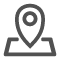# HBase数据表查询操作和获取多版本数据

HBase数据模型是一个多维稀疏映射。 HBase中的表包含列族，其列限定符包含值和时间戳。在大多数 HappyBase API 中，列族和限定符名称被指定为单个字符串，例如cf1:col1，而不是作为两个单独的参数。虽然列族和限定符在 HBase 数据模型中是不同的概念，但它们在与数据交互时几乎总是一起使用，因此将它们视为单个字符串会使 API 变得更简单。

## 查询行

Table 类提供了多种方法来从 HBase 中的表中检索数据。最基本的是 Table.row()，它从表中检索单行，并将其作为映射列到值的字典返回：

```row = table.row(b'rk0001')
print(row[b'info:name'])   # prints the value of info:name
# 返回值：b'zhangsan'，注意是二进制<class 'bytes'>类型
# 转换字符串：
print(str(row[b'info:name'], encoding="utf-8"))
# 即
str(bytes对象, encoding="编码格式，通常使用utf-8")```

> 注意，库中的字符串都有前缀：b，表示二进制

> 如：print(type(row[b'info:name']))

> 返回值：

## 查询多行

Table.rows() 方法的工作方式与 Table.row() 类似，但需要多个行键并将它们作为 (key, data) 元组返回：

```rows = table.rows([b'rk0001', b'rk0002'])
for key, data in rows:
print(key, data)

"""

b'rk0001' {b'data:pic': b'picture', b'info:age': b'20', b'info:gender': b'female', b'info:name': b'zhangsan'}
b'rk0002' {b'data:pic': b'picture', b'info:age': b'11', b'info:gender': b'male', b'info:name': b'wangwu'}

data是字典对象，字典中key和value都是bytes```

```rows_dict = dict(table.rows([b'rk0001', b'rk0002']))
# 返回值：
"""

{b'rk0001': {b'data:pic': b'picture', b'info:age': b'20',
b'info:gender': b'female', b'info:name': b'zhangsan'},
b'rk0002': {b'data:pic': b'picture', b'info:age': b'11',
b'info:gender': b'male', b'info:name': b'wangwu'}}
"""```

```from collections import OrderedDict
rows_as_ordered_dict = OrderedDict(table.rows([b'rk0002', b'rk0001']))

"""

OrderedDict([(b'rk0002', {b'data:pic': b'picture',
b'info:age': b'11', b'info:gender': b'male', b'info:name':
b'wangwu'}), (b'rk0001', {b'data:pic': b'picture',
b'info:age': b'20', b'info:gender': b'female',
b'info:name': b'zhangsan'})])
"""```

## 进行更细粒度的查询

HBase 的数据模型允许对要检索的数据进行更细粒度的选择。如果您事先知道需要哪些列，则可以通过将这些列明确指定给 Table.row() 和 Table.rows() 来提高性能。 columns 参数采用列名的列表(或元组)：

```row = table.row(b'rk0001', columns=[b'info:name', b'data:pic'])
# row对象类型字典，内容：{b'data:pic': b'picture', b'info:name': b'zhangsan'}
print(row[b'info:name'])
print(row[b'data:pic'])

"""

b'zhangsan'
b'picture'```

```row = table.row(b'rk0001', columns=[b'info'])
print(type(row))
print(row)

"""

<class 'dict'>
{b'info:age': b'20', b'info:gender': b'female', b'info:name': b'zhangsan'}
"""```

## 基于时间戳查询

`row = table.row(b'rk0001', timestamp=123456789)`

```row = table.row(b'rk0001', columns=[b'info'], include_timestamp=True)
print(type(row))
print(row)

"""

<class 'dict'>
{b'info:age': (b'20', 1641832837038), b'info:gender':
(b'female', 1641832832414), b'info:name': (b'zhangsan',
1641832826093)}
"""```

## 获取多版本数据

HBase 支持存储同一单元的多个版本。这可以为每个列族配置。要检索给定行的列的所有版本，可以使用 Table.cells()。此方法返回一个有序的单元格列表，最新版本排在最前面。版本参数指定要返回的最大版本数。就像检索行的方法一样，include_timestamp 参数确定时间戳是否包含在结果中。例子：

```# 首先，准备一个保存多版本的表
create 'tv', {NAME => 'info', VERSIONS => 5}
# 插入版本1
put 'tv', 'rk0001', 'info:name', 'hahaha'
# 插入版本2
put 'tv', 'rk0001', 'info:name', 'heiheihei'

# 检查
hbase(main):024:0> scan 'tv'
ROW                          COLUMN+CELL
rk0001                      column=info:name, timestamp=1641836267655, value=heiheihei
1 row(s)
Took 0.0103 seconds```
```table = connection.table('tv')
# 获取1个版本（默认最新咯）
values = table.cells(b'rk0001', b'info:name', versions=1)
print(type(values))
print(values)

"""

<class 'list'>
[b'heiheihei']
"""
# 获取2个版本
values = table.cells(b'rk0001', b'info:name', versions=2)
print(type(values))
print(values)

"""

<class 'list'>
[b'heiheihei', b'hahaha']

"""

# 获取多版本并附带时间戳信息
values = table.cells(b'rk0001', b'info:name', versions=2, include_timestamp=True)
print(type(values))
print(values)

"""

<class 'list'>
[(b'heiheihei', 1641836267655), (b'hahaha', 1641836263534)]
"""```